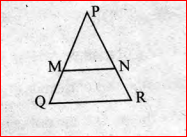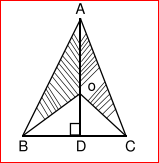# Kerala Class 9 Maths Important Questions

Mathematics is an important subject for all students. One might call it an abstract science of number, quantity or space, but students can also use abstraction and logic to authenticate it’s truth or even to devise its applicability in real life scenarios. Class 9 Maths is also of value as it provides students with a strong foundation in the subject, thus making it easier for them in class 10. Students who wish to score high in their Kerala Board Class 9, also consider Maths to be important. Meanwhile, most students also believe that maths subject can be very difficult and it requires a lot of practice. To provide students with the required practice of the sums as per the Kerala Syllabus 9th Standard, there are lots of study materials and useful resources available. Students access the textbooks and previous year question papers to get the required practice and also to self-assess their knowledge of a subject. The Kerala Board Class 9 Maths Important questions also comes in handy for these students.

### Benefits of Kerala Board Class 9 Maths Important Questions

• Covers important topics and concepts of the subject
• Students get practice answering important questions
• Help students to study for exams, as they are able to self-assess their knowledge gap
• Students gain confidence to face the final exams

Now, we have compiled some important questions here for the students to practice with. Shall we take a look?

Kerala Class 9 Maths Important Questions

1. (a)

$$\begin{array}{l}\frac{7}{3}+ \frac{7}{4}= \frac{28+21}{12}= \frac{49}{12}= \frac{7}{4} X \frac{7}{3}\end{array}$$

Write another pair of numbers with sum and product equal.

(b) If

$$\begin{array}{l}a+b=ab\end{array}$$
, then
$$\begin{array}{l}\frac{1}{a}+\frac{1}{b}\end{array}$$
=____________

2. In the figure, MN is parallel to QR. PM=6centimetres, PQ= 10 centimetres.

1. What is the length of MQ?2. What is PN:NR?

3. p(x)= x²- 3x +2, q(x)= 3x+1

1. Find p(x) + q(x)
2. If p(x)+q(x) + r(x) =0, find r(x).
3. What number is p(1) + q(1) + r(1)?

4. 10, 20, 30,———— are multiples are 10. They are in general represented as 10n, where n is any natural number. To get two digit multiple of 10, take the numbers from 1 to 9 for n. Similarly, the numbers, 11, 31, 21, —————-, 91 can generally be represented by 10n + 1. Here also n =1, 2,3,——-, 9.

Likewise, 12, 22, 32,———, is represented by 10n+ 2. In short all two digit numbers can be represented by 10n + m, where m= 1,2,3,4,———,9; m=0, 1,2,…………,9. Here n is a digit in Ten’s place and m is a digit in one’s place.

1. The digit in a ten’s place of a number is a and digit in it’s one’s place is b. What is the number?
2. Which is the number obtained by interchanging the digits in the number mentioned above?
3. Find the sum of the above two numbers.

5. David has a Recurring Deposit Account in a bank. He deposits Rs 2500 per month for 2 years. If he gets Rs, 66,250 at the time of maturity, find:

1. The interest paid by the bank
2. The rate of interest

6. Using a ruler and a compass, construct a parallelogram ABCD, given that AB=4cm, AC= 10 cm and BD= 6cm. Measure BC.

7. If the perimeter of a rectangular plot is 68m and the length of its diagonal is 26m. Find it’s area.

8. A car covers a distance of 400km at a certain speed. Had the speed been 12km/hr or more, the time taken for the journey would have been 1 hour 40 minutes less. Find the original speed of the car.

9. Rationalise

$$\begin{array}{l}\frac{2}{\sqrt{5} +\sqrt{3}+\sqrt{2}}\end{array}$$

10. Solve for x and y. ‘

41x + 53y = 135

53x+ 41y= 147

11. If

$$\begin{array}{l}\frac{Sec\theta + tan \theta}{Sec \theta – tan \theta}=\frac{4}{1}\end{array}$$
,then Sin

12. Six years hence a man’s age will be three times his son’s age and three years ago he was nine times as old as his son. Find their present ages.

13. Construct a parallelogram with diagonals 6 cm and 8cm in length and angle between them is 60º. Measure a longer side.

14. Construct an equilateral triangle of side 5 cm and draw its incircle.

15. Find the mean, median and mode of 8,0,5,3, 2,2,1,2,4, 7, 2, 5.

16. What sum will amount to Rs 2782.50 in 2 years at CI, if the rates are 5% and 5% for the successive years?

17. Find the coordinates of the center of a circle passing through A(5,1), B(-3, -7) and C(7,-1).

18. The result of dividing a number of two digits by the number with the digits reversed is ⅚. If the difference of the digits is 1, find the number.

19. In the adjoining figure, ABC is an isosceles triangle with BC=8cm, AB=AC=12cm. AD is perpendicular to BC and O is a point of AB such that <BOC=90º, find the area of shaded portion.20. How many square tiles of side 20cm will be needed to pave a footpath, which is 2m wide and surrounds a rectangular plot 40m by 22m.